Math Skills ReviewSignificant Figures

There are two kinds of numbers in the world:

• exact:
• example: There are exactly 12 eggs in a dozen.
• example: Most people have exactly 10 fingers and 10 toes.
• inexact numbers:
• example: any measurement.
If I quickly measure the width of a piece of notebook paper, I might get 220 mm (2 significant figures). If I am more precise, I might get 216 mm (3 significant figures). An even more precise measurement would be 215.6 mm (4 significant figures).

PRECISION VERSUS ACCURACY

Accuracy refers to how closely a measured value agrees with the correct value.
Precision refers to how closely individual measurements agree with each other.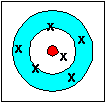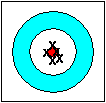accurate(the average is accurate)not precise precisenot accurate accurateandprecise

In any measurement, the number of significant figures is critical. The number of significant figures is the number of digits believed to be correct by the person doing the measuring. It includes one estimated digit. So, does the concept of significant figures deal with precision or accuracy? I'll answer this question after you peruse the next example.

Let's look at an example where significant figures is important: measuring volume in the laboratory. This can be done in many ways: using

• a beaker with volumes marked on the side,
• a buret.
Which glassware would give you the most precise volume measurement? Let's figure out the volume for each one and its associated error. This will give us the number of figures that are significant. Recall: the number of significant figures includes one estimated digit.

A rule of thumb: read the volume to 1/10 or 0.1 of the smallest division. (This rule applies to any measurement.) This means that the error in reading (called the reading error) is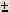1/10 or 0.1 of the smallest division on the glassware. If you are less sure of yourself, you can read to 1/5 or 0.2 of the smallest division.

 Beaker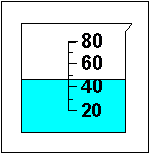The smallest division is 10 mL, so we can read the volume to1/10 of 10 mL or1 mL. The volume we read from the beaker has a reading error of1 mL.The volume in this beaker is 471 mL. You might have read 46 mL; your friend might read the volume as 48 mL. All the answers are correct within the reading error of1 mL.So, How many significant figures does our volume of 471 mL have? Answer - 2! The "4" we know for sure plus the "7" we had to estimate. GraduatedCylinder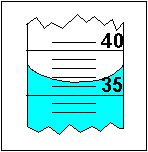Look in the textbook for a picture of a graduated cylinder. First, note that the surface of the liquid is curved. This is called the meniscus. This phenomenon is caused by the fact that water molecules are more attracted to glass than to each other (adhesive forces are stronger than cohesive forces). When we read the volume, we read it at the BOTTOM of the meniscus.The smallest division of this graduated cylinder is 1 mL. Therefore, our reading error will be0.1 mL or 1/10 of the smallest division. An appropriate reading of the volume is 36.50.1 mL. An equally precise value would be 36.6 mL or 36.4 mL. How many significant figures does our answer have? 3! The "3" and the "6" we know for sure and the "5" we had to estimate a little. Buret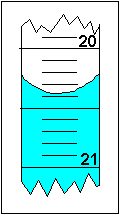Look in the textbook for a picture of a buret. Note that the numbers get bigger as you go down the buret. This is different from the beaker or the graduated cylinder. This is because the liquid leaves the buret at the bottom. The smallest division in this buret is 0.1 mL. Therefore, our reading error is0.01 mL. A good volume reading is 20.380.01 mL. An equally precise answer would be 20.39 mL or 20.37 mL. How many significant figures does our answer have? 4! The "2", "0", and "3" we definitely know and the "8" we had to estimate.

Conclusion: The number of significant figures is directly linked to a measurement. If a person needed only a rough estimate of volume, the beaker volume is satisfactory (2 significant figures), otherwise one should use the graduated cylinder (3 significant figures) or better yet, the buret (4 significant figures).

So, does the concept of significant figures deal with precision or accuracy? Hopefully, you can see that it really deals with precision only. Consider measuring the length of a metal rod several times with a ruler. You will get essentially the same measurement over and over again with a small reading error equal to about 1/10 of the smallest division on the ruler. You have determined the length with high precision. However, you don't know if the ruler was accurate to begin with. Perhaps it was a plastic ruler left in the hot Texas sun and was stretched. You don't know the accuracy of your measuring device unless you calibrate it, i.e. compare it against a ruler you knew was accurate. Note: in the laboratory, a good analytical chemist always calibrates her volumetric glassware before using it by weighing a known volume of liquid dispensed from the glassware. By dividing the mass of the liquid by its density, she can determine the actual volume and hence the accuracy of the glassware.

Rules for Working with Significant Figures:

1. Leading zeros are never significant.
Imbedded zeros are always significant.
Trailing zeros are significant only if the decimal point is specified.
Hint: Change the number to scientific notation. It is easier to see.

The last digit retained is set by the first doubtful digit.

3. Multiplication or Division:
The answer contains no more significant figures than the least accurately known number.

EXAMPLES:

 Example Number ofSignificant Figures Scientific Notation 0.00682 3 6.82 x 10-3 Leading zeros are not significant. 1.072 4 1.072 (x 100) Imbedded zeros are always significant. 300 1 3 x 102 Trailing zeros are significant only if the decimal point is specified. 300. 3 3.00 x 102 300.0 4 3.000 x 102

EXAMPLES
 Addition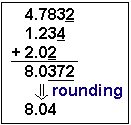Even though your calculator gives you the answer 8.0372, you must round off to 8.04. Your answer must only contain 1 doubtful number. Note that the doubtful digits are underlined. Subtraction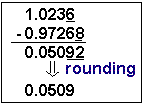Subtraction is interesting when concerned with significant figures. Even though both numbers involved in the subtraction have 5 significant figures, the answer only has 3 significant figures when rounded correctly. Remember, the answer must only have 1 doubtful digit. Multiplication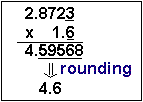The answer must be rounded off to 2 significant figures, since 1.6 only has 2 significant figures. Division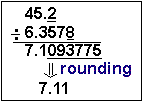The answer must be rounded off to 3 significant figures, since 45.2 has only 3 significant figures.

Notes on Rounding

• When rounding off numbers to a certain number of significant figures, do so to the nearest value.
• example: Round to 3 significant figures: 2.3467 x 104 (Answer: 2.35 x 104)
• example: Round to 2 significant figures: 1.612 x 103 (Answer: 1.6 x 103)

• What happens if there is a 5? There is an arbitrary rule:
• If the number before the 5 is odd, round up.
• If the number before the 5 is even, let it be.
The justification for this is that in the course of a series of many calculations, any rounding errors will be averaged out.

• example: Round to 2 significant figures: 2.35 x 102 (Answer: 2.4 x 102)
• example: Round to 2 significant figures: 2.45 x 102 (Answer: 2.4 x 102)

• Of course, if we round to 2 significant figures: 2.451 x 102, the answer is definitely 2.5 x 102 since 2.451 x 102 is closer to 2.5 x 102 than 2.4 x 102.

QUIZ:
 Question 1 Give the correct number of significant figures for 4500, 4500., 0.0032, 0.04050 Question 2 Give the answer to the correct number of significant figures: 4503 + 34.90 + 550 = ? Question 3 Give the answer to the correct number of significant figures: 1.367 - 1.34 = ? Question 4 Give the answer to the correct number of significant figures: (1.3 x 103)(5.724 x 104) = ? Question 5 Give the answer to the correct number of significant figures: (6305)/(0.010) = ?

Answers: (1) 2, 4, 2, 4 (2) 5090 (3 significant figures - round to the tens place - set by 550) (3) 0.03 (1 significant figure - round to hundredths place) (4) 7.4 x 107 (2 significant figures - set by 1.3 x 103) (5) 6.3 x 105 (2 significant figures - set by 0.010)Algebraic ManipulationScientific NotationManipulation of ExponentsDimensional AnalysisLogarithmsThe Quadratic Equation